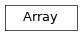# np_conserved¶

Classes`Array`(legcharges[, dtype, qtotal, labels]) A multidimensional array (=tensor) for using charge conservation.

Functions

 `concatenate`(arrays[, axis, copy]) Stack arrays along a given axis, similar as np.concatenate. `detect_grid_outer_legcharge`(grid, grid_legs) Derive a LegCharge for a grid used for `grid_outer()`. `detect_legcharge`(flat_array, chargeinfo, ...) Calculate a missing LegCharge by looking for nonzero entries of a flat array. `detect_qtotal`(flat_array, legcharges[, cutoff]) Returns the total charge (w.r.t legs) of the sector with largest entry in flat_array. `diag`(s, leg[, dtype, labels]) Returns a square, diagonal matrix of entries s. `eig`(a[, sort]) Calculate eigenvalues and eigenvectors for a non-hermitian matrix. `eigh`(a[, UPLO, sort]) Calculate eigenvalues and eigenvectors for a hermitian matrix. `eigvals`(a[, sort]) Calculate eigenvalues for a hermitian matrix. `eigvalsh`(a[, UPLO, sort]) Calculate eigenvalues for a hermitian matrix. Use scipy.linalg.expm to calculate the matrix exponential of a square matrix. `eye_like`(a[, axis, labels]) Return an identity matrix contractible with the leg axis of the `Array` a. `grid_concat`(grid, axes[, copy]) Given an np.array of npc.Arrays, performs a multi-dimensional concatentation along 'axes'. `grid_outer`(grid, grid_legs[, qtotal, ...]) Given an np.array of npc.Arrays, return the corresponding higher-dimensional Array. `inner`(a, b[, axes, do_conj]) Contract all legs in a and b, return scalar. `norm`(a[, ord, convert_to_float]) Norm of flattened data. `ones`(legcharges[, dtype, qtotal, labels]) Short-hand for `Array.from_func()` with function `numpy.ones()`. `orthogonal_columns`(a[, new_label]) Find orthogonal columns for a given matrix. `outer`(a, b) Forms the outer tensor product, equivalent to `tensordot(a, b, axes=0)`. `pinv`(a[, cutoff]) Compute the (Moore-Penrose) pseudo-inverse of a matrix. `qr`(a[, mode, inner_labels, cutoff, ...]) Q-R decomposition of a matrix. `speigs`(a, charge_sector, k, *args, **kwargs) Sparse eigenvalue decomposition `w, v` of square a in a given charge sector. `svd`(a[, full_matrices, compute_uv, cutoff, ...]) Singualar value decomposition of an Array a. `tensordot`(a, b[, axes]) Similar as `np.tensordot` but for `Array`. `to_iterable_arrays`(array_list) Similar as `to_iterable()`, but also enclose npc Arrays in a list. `trace`(a[, leg1, leg2]) Trace of a, summing over leg1 and leg2. `zeros`(legcharges[, dtype, qtotal, labels]) Create a npc array full of zeros (with no _data).

Module description

A module to handle charge conservation in tensor networks.

A detailed introduction to this module (including notations) can be found in Charge conservation with np_conserved.

This module np_conserved implements a class `Array` designed to make use of charge conservation in tensor networks. The idea is that the Array class is used in a fashion very similar to the `numpy.ndarray`, e.g you can call the functions `tensordot()` or `svd()` (of this module) on them. The structure of the algorithms (as DMRG) is thus the same as with basic numpy ndarrays.

Internally, an `Array` saves charge meta data to keep track of blocks which are nonzero. All possible operations (e.g. tensordot, svd, …) on such arrays preserve the total charge structure. In addition, these operations make use of the charges to figure out which of the blocks it has to use/combine - this is the basis for the speed-up.

tenpy.linalg.np_conserved.QCUTOFF = 2.220446049250313e-15

A cutoff to ignore machine precision rounding errors when determining charges

tenpy.linalg.np_conserved.QTYPE = <class 'numpy.int64'>

the type used for charges

## Overview¶

Classes

 `Array`(legcharges[, dtype, qtotal, labels]) A multidimensional array (=tensor) for using charge conservation. `ChargeInfo`([mod, names]) Meta-data about the charge of a tensor. `LegCharge`(chargeinfo, slices, charges[, qconj]) Save the charge data associated to a leg of a tensor. `LegPipe`(legs[, qconj, sort, bunch]) A LegPipe combines multiple legs of a tensor to one.

Array creation

 `Array.from_ndarray_trivial`(data_flat[, ...]) convert a flat numpy ndarray to an Array with trivial charge conservation. `Array.from_ndarray`(data_flat, legcharges[, ...]) convert a flat (numpy) ndarray to an Array. `Array.from_func`(func, legcharges[, dtype, ...]) Create an Array from a numpy func. `Array.from_func_square`(func, leg[, dtype, ...]) Create an Array from a (numpy) function. `zeros`(legcharges[, dtype, qtotal, labels]) Create a npc array full of zeros (with no _data). `eye_like`(a[, axis, labels]) Return an identity matrix contractible with the leg axis of the `Array` a. `diag`(s, leg[, dtype, labels]) Returns a square, diagonal matrix of entries s.

Concatenation

 `concatenate`(arrays[, axis, copy]) Stack arrays along a given axis, similar as np.concatenate. `grid_concat`(grid, axes[, copy]) Given an np.array of npc.Arrays, performs a multi-dimensional concatentation along 'axes'. `grid_outer`(grid, grid_legs[, qtotal, ...]) Given an np.array of npc.Arrays, return the corresponding higher-dimensional Array.

Detecting charges of flat arrays

 `detect_qtotal`(flat_array, legcharges[, cutoff]) Returns the total charge (w.r.t legs) of the sector with largest entry in flat_array. `detect_legcharge`(flat_array, chargeinfo, ...) Calculate a missing LegCharge by looking for nonzero entries of a flat array. `detect_grid_outer_legcharge`(grid, grid_legs) Derive a LegCharge for a grid used for `grid_outer()`.

Contraction of some legs

 `tensordot`(a, b[, axes]) Similar as `np.tensordot` but for `Array`. `outer`(a, b) Forms the outer tensor product, equivalent to `tensordot(a, b, axes=0)`. `inner`(a, b[, axes, do_conj]) Contract all legs in a and b, return scalar. `trace`(a[, leg1, leg2]) Trace of a, summing over leg1 and leg2.

Linear algebra

 `svd`(a[, full_matrices, compute_uv, cutoff, ...]) Singualar value decomposition of an Array a. `pinv`(a[, cutoff]) Compute the (Moore-Penrose) pseudo-inverse of a matrix. `norm`(a[, ord, convert_to_float]) Norm of flattened data. `qr`(a[, mode, inner_labels, cutoff, ...]) Q-R decomposition of a matrix. Use scipy.linalg.expm to calculate the matrix exponential of a square matrix.

Eigen systems

 `eigh`(a[, UPLO, sort]) Calculate eigenvalues and eigenvectors for a hermitian matrix. `eig`(a[, sort]) Calculate eigenvalues and eigenvectors for a non-hermitian matrix. `eigvalsh`(a[, UPLO, sort]) Calculate eigenvalues for a hermitian matrix. `eigvals`(a[, sort]) Calculate eigenvalues for a hermitian matrix. `speigs`(a, charge_sector, k, *args, **kwargs) Sparse eigenvalue decomposition `w, v` of square a in a given charge sector.